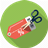DiscountQuantitative Aptitude Questions and Answers section on “Discount” with solution and explanation for competitive examinations such as CAT, MBA, SSC, Bank PO, Bank Clerical and other examinations.

Two successive discounts of 10% and 5% are equivalent to a single discount of :

Two successive discounts of 10% and 5% are equivalent to a single discount of : [A]14% [B]14.25% [C]14.50% [D]15% Show Answer 14.50% Single equivalent discount = $latex = \left ( 10+5-\frac{10\times 5}{100} \right )\% = 14.5\%&s=1$ Hence option [C] is correct answer.

Two successive discounts of 20% and 20% are equivalent to a single discount of :

Two successive discounts of 20% and 20% are equivalent to a single discount of : [A]34% [B]36% [C]40% [D]42% Show Answer 36% Single equivalent discount = $latex = \left ( 20+20-\frac{20\times 20}{100} \right )\% = 36\%&s=1$ Hence option [B] is correct answer.

What single discount is equivalent to two successive discounts of 20% and 15%?

What single discount is equivalent to two successive discounts of 20% and 15%? [A]30% [B]32% [C]34% [D]35% Show Answer 32% Single equivalent discount = $latex = \left ( x+y-\frac{x\times y}{100} \right )\%&s=1$ $latex = \left ( 20+15-\frac{20\times 15}{100} \right )\% = 32\%&s=1$ Hence option [B] is correct answer.